# Category Archives: Channel Modeling

Rayleigh fading channel and its various implementations.

# Reflection vs Scattering in Ray-Tracing

In ray-tracing simulations one is faced with a complexity at points of intersection between rays and objects. How to handle the reflection, should it be specular or scattered. In this post we consider the two extreme cases; pure reflection vs scattering.

For this we consider that in the case of specular reflection the power of the ray is reduced by 3dB (that is R=0.5) and the ray continues as it would if there was no interaction with the object (off course direction would be changed with angle of reflection being equal to angle of incidence).

In the case of scattered ray we assume that the point of interaction between the ray and the object is a point source from where the rays are regenerated. Therefore there is a rapid drop in the E-field strength as the ray propagates away from the point of interaction.

```clear all
close all

Eo=1;
r1=1:100;
r2=101:200;
r=[r1 r2];
R=0.5;

Er1=Eo./r1;
Er2=R*Eo./r2;
Er=[Er1 Er2];

Es1=Eo./r1;
Es2=Es1(end)./(r2-r1(end));
Es=[Es1 Es2];

plot(r,10*log10(Er),'b')
hold on
plot(r,10*log10(Es),'r:')
hold off

legend('Reflection','Scattering')
xlabel('Distance (m)')
ylabel('E-field (V/m)')
```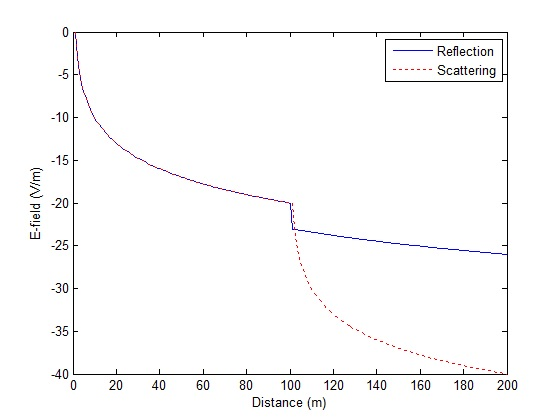The simulation code above considers that a ray travels unobstructed for 100 m and at this point comes in contact with an object and is reflected (reflection coefficient of 0.5 is assumed) or scattered. The ray then again travels for another 100 m without coming in contact with an object.

It is observed that there is a rapid decrease in E-field strength of the scattered ray beyond 100 m. This is because the E-field strength at the point of interaction is much lower than that at the source and when this is considered to be a point source, re-generating the rays, the resulting E-field decays quite rapidly.

We assume that the reflection is specular in most of our simulations as this is closer to reality than assuming a fully scattered ray.

# Eclipse

#### Eclipse 1.0 – A Paradigm Shift in RF Planning

NEW: Simulation of a Moving Transmitter (such as a car)

NEW: Simulation of a Moving Transmitter (such as a pedestrian)

Radio frequency planning is an essential component of network planning, roll-out, up-gradation, expansion etc. Several methods can be adopted for this from something as simple as free space models, empirical path loss models to the significantly more complicated, time consuming and expensive drive testing. Drive testing gives very accurate results but these results can be rendered useless by changing the position of an antenna or the tilt or transmit power of an antenna requiring another run in the field. One solution to this problem is ray-tracing which is very accurate but is usually considered to be very computationally expensive and of little practical value. But recent advances in computational power of machines coupled with efficient techniques have given a new lease of life to this method.

Eclipse is a near real-time simulation software for prediction of signal strength in urban areas. The software uses shooting and bouncing ray (SBR) method of ray tracing with 1 degree ray separation, 1 m step size and 9 interactions per ray path. The simulation parameters can be varied according to the resolution required. The code is highly optimized to give results in shortest possible time. It is especially useful for network planning of ultra-dense wireless networks where a dense network of antennas is placed on lamp posts instead of telecom towers. Various frequency bands can be simulated, along with different antenna radiation patterns and MIMO configurations.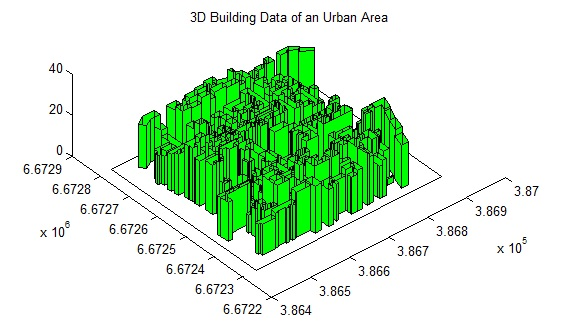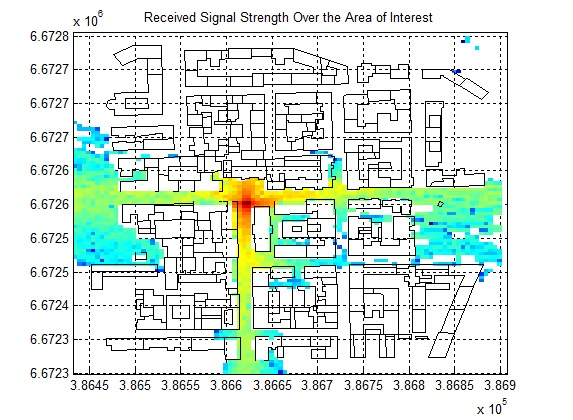Note: If you would like to run a test simulation send us a request at info@raymaps.com

# Sum of Sinusoids Fading Simulator

We have previously looked at frequency domain fading simulators i.e. simulators that define the Doppler components in the frequency domain and then perform an IDFT to get the time domain signal. These simulators include Smith’s Simulator, Young’s Simulator and our very own Computationally Efficient Rayleigh Fading Simulator. Another technique that has been widely reported in the literature is Sum of Sinusoids Method. As the name suggests this method generates the Doppler components in the time domain and then sums them up to generate the time domain fading envelope. There are three parameters that define the properties of the generated signal.

1) Number of sinusoids – Higher the number better the properties of the generated signal but greater the computational complexity
2) Angle of arrival – This can be generated statistically or deterministically, spread from –pi to pi.
3) Phase of the arriving wave – This is uniformly distributed between –pi and pi.

The MATLAB code below gives three similar sum of sinusoids techniques for generating a Rayleigh faded envelope .

```%%%%%%%%%%%%%%%%%%%%%%%%%%%%%%%%%%%%%%%%%%%%%
% SUM OF SINUSOIDS FADING SIMULATORS
% fd - Doppler frequency
% fs - Sampling frequency
% ts - Sampling period
% N - Number of sinusoids
%
% www.raymaps.com
%%%%%%%%%%%%%%%%%%%%%%%%%%%%%%%%%%%%%%%%%%%%%

clear all
close all

fd=70;
fs=1000000;
ts=1/fs;
t=0:ts:1;
N=100;

%%%%%%%%%%%%%%%%%%%%%%%%%%%%%%%%%%%%%%%%%%%%%
% Method 1 - Clarke
%%%%%%%%%%%%%%%%%%%%%%%%%%%%%%%%%%%%%%%%%%%%%
x=zeros(1,length(t));
y=zeros(1,length(t));

for n=1:N;n
alpha=(rand-0.5)*2*pi;
phi=(rand-0.5)*2*pi;
x=x+randn*cos(2*pi*fd*t*cos(alpha)+phi);
y=y+randn*sin(2*pi*fd*t*cos(alpha)+phi);
end
z=(1/sqrt(N))*(x+1i*y);
r1=abs(z);

plot(t,10*log10(r1))
hold on

%%%%%%%%%%%%%%%%%%%%%%%%%%%%%%%%%%%%%%%%%%%%%
% Method 2 - Pop, Beaulieu
%%%%%%%%%%%%%%%%%%%%%%%%%%%%%%%%%%%%%%%%%%%%%
x=zeros(1,length(t));
y=zeros(1,length(t));

for n=1:N;n
alpha=2*pi*n/N;
phi=(rand-0.5)*2*pi;
x=x+randn*cos(2*pi*fd*t*cos(alpha)+phi);
y=y+randn*sin(2*pi*fd*t*cos(alpha)+phi);
end
z=(1/sqrt(N))*(x+1i*y);
r2=abs(z);

plot(t,10*log10(r2),'r')
hold on

%%%%%%%%%%%%%%%%%%%%%%%%%%%%%%%%%%%%%%%%%%%%%
% Method 3 - Chengshan Xiao
%%%%%%%%%%%%%%%%%%%%%%%%%%%%%%%%%%%%%%%%%%%%%
x=zeros(1,length(t));
y=zeros(1,length(t));

for n=1:N;n
phi=(rand-0.5)*2*pi;
theta=(rand-0.5)*2*pi;
alpha=(2*pi*n+theta)/N;
x=x+randn*cos(2*pi*fd*t*cos(alpha)+phi);
y=y+randn*sin(2*pi*fd*t*cos(alpha)+phi);
end
z=(1/sqrt(N))*(x+1i*y);
r3=abs(z);

plot(t,10*log10(r3),'g')
hold off

xlabel('Time(sec)')
ylabel('Envelope(dB)')
%%%%%%%%%%%%%%%%%%%%%%%%%%%%%%%%%%%%%%%%%%%%%
```

All the three techniques given above are quite accurate in generating a Rayleigh faded envelope with the desired statistical properties. The accuracy of these techniques increases as the number of sinusoids goes to infinity (we have tested these techniques with up to 1000 sinusoids but realistically speaking even 100 sinusoids are enough). If we want to compare the three techniques in terms of the Level Crossing Rate (LCR) and Average Fade Duration (AFD) we can say that the first and third technique are a bit more accurate than the second technique. Therefore we can conclude that a statistically distributed angle of arrival is a better choice than a deterministically distributed angle of arrival. Also, if we look at the autocorrelation of the in-phase and quadrature components we see that for the first and third case we get a zero order Bessel function of the first kind whereas for the second case we get a somewhat different sequence which approximates the Bessel function with increasing accuracy as the number of sinusoids is increased.

The above figures show the theoretical Bessel function versus the autocorrelation of the real/imaginary part  generated by method number two. The figure on the left considers 20 sinusoids whereas the figure on the right considers 40 sinusoids. As can be seen the accuracy of the autocorrelation sequence increases considerably by doubling the number of sinusoids. We can assume that for number of sinusoids exceeding 100 i.e. N=100 in the above code the generated autocorrelation sequence would be quite accurate.

 Chengshan Xiao, “Novel Sum-of-Sinusoids Simulation Models for Rayleigh and Rician Fading Channels,” IEEE TRANSACTIONS ON WIRELESS COMMUNICATIONS, VOL. 5, NO. 12, DECEMBER 2006.

In the previous posts we had discussed generation of a correlated Rayleigh fading sequence using Smith’s method  and Young’s modification of Smith’s method . The main contribution of Young was that he proposed a mechanism where the number of IDFTs was reduced by half. This was achieved by first adding two length N IID zero mean Gaussian sequences filtered by the filter F[k] and then performing the IDFT on the resulting complex sequence.

This was different to Smith’s method where the IDFT was performed simultaneously on two branches and then the outputs of these branches were added in quadrature to achieve the desired sequence with Rayleigh distributed envelope and Uniformly distributed phase. Another problem with Smith’s method was that the outputs of the two arms after performing IDFTs was assumed to be real which is not always the case in implementation and depends upon the combination of Doppler frequency (fm) and length of Gaussian sequence (N).

Young’s technique is shown graphically in the above figure. Also shown is the definition of filter F[k] which depends upon N, fm and km (please note that the fm in the above equation is normalized by the sampling frequency fs). Here km = N*(fm/fs). We propose three modifications to Young’s technique which significantly reduces computation and at the same time maintains the statistical properties of the generated sequence. The modifications we propose are.

1. First modification has to do with the generated Gaussian sequence. It is observed that the filter F[k], at very high sampling rates, is mostly zero and there are very few points which have some non-zero value. So when we multiply the Gaussian sequence with the filter we mostly get zeros at the output. So we propose that the filter response in the frequency domain must be calculated first and the the Gaussian random sequence must be generated for only those points where the filter F[k] is non-zero e.g. for a sampling frequency of 7.68 MHz (standard sampling frequency for a BW of 5 MHz in LTE) and Doppler frequency of 70 Hz (corresponding to medium Doppler case in LTE) the filter F[k] has 99.9982% zeros in its frequency response and it would be a highly wasteful to calculate Gaussian RVs for all those values.

2. Secondly according to Clarke  the Doppler Spectrum measurements have “Marked disagreement at very low frequencies and at frequencies in the region of the sharp cut-off associated with the maximum Doppler frequency shift. At very low frequencies the spectral energy is always observed to be higher than that predicted by theory”. He goes on to add “The reason for this is that neither theoretical model takes into account the large scale variations in total energy which result from the changing topography between transmitter and mobile receiver”. This suggests that the Classical Doppler Spectrum might not be the best choice under all scenarios. This has also been noted in  where a flat fading model is evaluated in terms of its Level Crossing Rate and Average Fade Duration. Such a flat spectrum is especially suited to indoor scenarios as noted in  and .

We propose a filter that gives equal weight to all the frequencies up to the maximum Doppler frequency. So our filter is a box-type filter which applies a constant scaling factor to all the frequencies in the pass-band and zeros out all the frequencies in the stop-band. So in fact the Gaussian sequence that is generated in the in-phase arm may directly be added with the Gaussian sequence from the other arm without applying the frequency domain filter and then IDFT of the complex sequence is taken. We will look into the deviation from ideality  that this causes later.

3. The third modification that we propose is in the implementation of the IDFT. Here again we take into consideration that the complex sequence being fed to the IDFT is filled with zeros (as we noted earlier 99.9982% zeros for 7.68 MHz and even more for higher frequencies) so we can avoid a lot multiplications and summations. The IDFT is defined below and also given is our modification to it.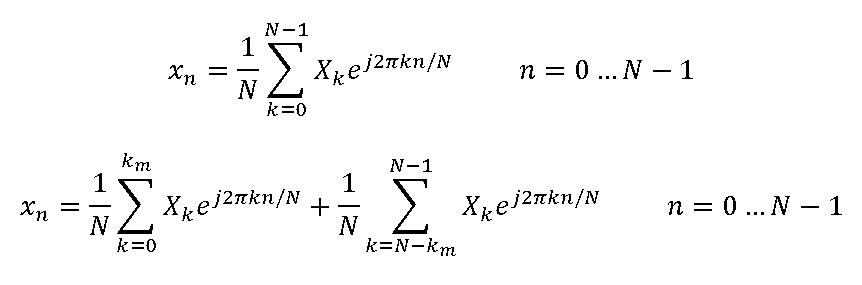Further improvement in computation time is achieved by implementing the above as a matrix multiplication. The matrix multiplication is implemented as H*X where H is the IDFT coefficients of size N x 2(km+1) and X is a vector of size 2(km+1) x 1 upon which the IDFT has to be performed.

Now let us look at the output sequence generated by using the above techniques. We consider the case of Medium Doppler Frequency of 70 Hz (EVA channel) as defined by LTE specifications. Sampling frequency is fixed at 10 kHz giving a normalized Doppler frequency of 0.007.  This was done due to limitation of memory on the machine. The author also experimented with a sampling frequency of 7.68 MHz but this did not yield enough samples for statistically accurate results. We did use a sampling frequency of 7.68 MHz for our bit error rate simulation which is shown in the end.

It is observed for fm=70 Hz the envelope of the output sequence using the proposed technique matches quite well with the envelope of the output sequence generated by the ideal filter proposed by Young. Also the phase and envelope of the sequence generated using the proposed technique has the desired distribution. Some of the other metrics that we can look at are the level crossing rate (LCR) and average fade duration (AFD) as well as the Auto Correlation of the real and imaginary parts of the complex sequence generated.

 Parameter Young Modified LCR (ideal) 48.1086 48.1086 LCR (sim) 48.1506 39.4348 AFD (ideal) 0.0018 0.0018 AFD (sim) 0.0018 0.0022

If we look at the results for LCR and AFD we see that the simulated results match reasonably well with the results predicted by theory. These results correspond to 100 snapshots of the fading sequence. It was important to take the average of several snapshots as results varied with each simulation run. Sometimes Young’s technique produced more accurate results while at other times the proposed technique was better. Again the limitations of computer memory and processing power dictated the length of the sequence that could be generated.

In general Young’s technique produced better results than our proposed technique. It was found that product of LCR and AFD for both cases matched quite well with the theoretical value. So the total time spent in a fade state per second was equal in both the cases. In the proposed method the duration of a single fading event was higher,  whereas the number of fading events per second was lower. This can be attributed to the fact that in our proposed technique higher weighting is given to lower frequency components and the fading sequence is smoothed out by these low frequency components. One technique to overcome this is spectral broadening as suggested by  but this is not the subject here and we postpone its discussion to another article.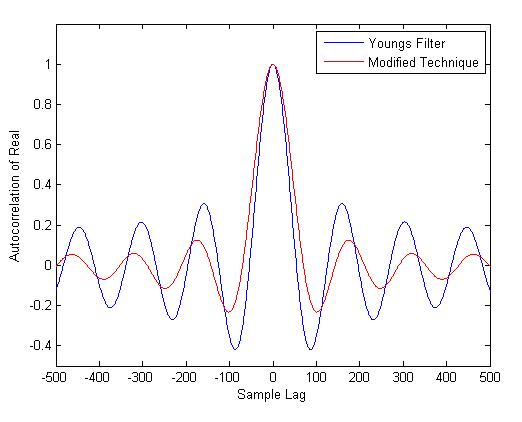Auto Correlation of Real Part fm=70Hz

The Auto Correlation of the real and imaginary parts of the generated sequences are also calculated for a Doppler frequency of 70 Hz. It is found that the Auto Correlation sequence for the two techniques matches quite well. However, the Auto Correlation sequence deviates from the theoretical value as calculated the by Bessel function of the first kind and zero order. Since we have used a flat spectral mask the Auto Correlation function resembles the sinc(x) function which is the same as zeroth order Spherical Bessel Function of the first kind (which is related to 1/2 order Bessel Function of the first kind).

It was found that when the Rayleigh fading sequence is generated by the program provided in Young’s thesis the shape of the Auto Correlation function depends upon the sampling frequency. At a normalized Doppler frequency of 0.05 and N=2^16 Young’s technique produces quite accurate results. We also measured the mean squared error (MSE) between the two Auto Correlations sequences and found it to be a function of the Normalized Doppler Frequency. It was found that as the Normalized Doppler Frequency was increased from 0.00007 to 0.0007 the MSE error dropped from 0.0277 to 0.0041. The relationship between the Normalized Doppler Frequency and MSE, for a fixed sequence length, seems to be resembling an exponential function. For more accurate results, at higher sampling frequencies, the number of samples would have to be increased considerably. In fact it was found that if the variable km (km=N*fm/fs) is maintained at around 20 the error between the two correlation sequences is less than 1% for all possible sampling rates.

We also compared the bit error rate (BER) performance of different QAM modulation schemes using both the techniques for fading envelope generation and found these to be matching quite well. A single tap was used which results in a flat fading channel. This is a simplistic channel model but it gives us some confidence that the proposed approach does have the desired statistical properties. A good test of a temporally correlated Rayleigh fading sequence is to test it on a system that implements interleavers and channel coders whose performance strongly depends upon factors such as the LCR and AFD e.g. a certain forward error correction (FEC) code might work well in high LCR and low AFD as this distributes out errors in different blocks and allows the code to correct them. In simulations done so far (not shown here) we have found that for a 1/2 rate convolutional encoder with Hard Viterbi Decoding the BER for the two schemes matches quite well. In general the results for correlated fading are much worse than uncorrelated fading.

In future we would also like to evaluate the bit error rate (BER) performance of an M-QAM OFDM system with Frequency Selective Rayleigh fading as described by the LTE fading channels EPA, EVA and ETU. This is probably a good scenario to compare the accuracy of the two techniques used to generate Rayleigh fading sequences above. One challenge in this regard is that the LTE channel taps are described in increments of 10 nsec whereas the LTE signal sampling rate is defined on a different scale (minimum Ts=32.5521 nsec corresponding to a sampling rate of 30.72 Msps). So we would have to do sample rate conversion to implement a time varying frequency selective Rayleigh fading channel.

 John I. Smith, “A Computer Generated Multipath Fading Simulation for Mobile Radio”, IEEE Transactions on Vehicular Technology, vol VT-24, No. 3, August 1975.

 David J. Young and Norman C. Beaulieu, “The Generation of Correlated Rayleigh Random Variates by Inverse Discrete Fourier Transform”, IEEE Transactions on Communications vol. 48 no. 7 July 2000.

 R. H. Clarke, A Statistical Theory of Mobile Radio Reception”, Bell Systems Technical Journal 47 (6), pp 957–1000, July 1968.

 Rosmansyah, Y.; Saunders, S.R.; Sweeney, P.; Tafazolli, R., “Equivalence of flat and classical Doppler sample generators,” Electronics Letters , vol.37, no.4, pp.243,244, 15 Feb 2001.

 JTC (Joint Technical Committee T1 RIP1.4 and TIA TR46.3.3/TR45.4.4 on Wireless Access): “Draft final report on RF channel characterization”. Paper no. JTC(AIR)/94.01.17-238R4, January 17, 1994.

 ETSI (European Telecommunications Standards Institute): “Universal mobile telecommunications system (UMTS); selection procedures for the choice of radio transmission technologies of the UMTS (UMTS 30.03 version 3.2.0)”. Technical Report, TR 101 112 V3.2.0 (1998-04), http://www.etsi.org, 1998.

# Uniform, Gaussian and Rayleigh Distribution

It is sometimes important to know the relationship between various distributions. This can be useful if there is a function available for one distribution and it can be used to derive other distributions. In the context of Wireless Communications it is important to know the relationship between the Uniform, Gaussian and Rayleigh distribution.

According to Central Limit Theorem the sum of a large number of independent and identically distributed random variables has a Gaussian distribution. This is used to model the amplitude of the in-phase and quadrature components of a wireless signal. Shown below is the model for the received signal which has been modulated by the Gaussian channel coefficients g1 and g2.

r=g1*a1*cos(2*pi*fc*t)+g2*a2*sin(2*pi*fc*t)

The envelope of this signal (sqrt(g1^2+g2^2)) as a Rayleigh distribution. Now if you only had a function for Uniform Distribution you can generate Rayleigh Distribution using the following routine.

```clear all
close all
M=10000;
N=100;

for n=1:M;
x1=rand(1,N)-0.5;
x2=rand(1,N)-0.5;

y1=mean(x1);
y2=mean(x2);

z(n)=sqrt(y1^2+y2^2);
end

hist(z,20)```

Note: Here a1 and a2 can be considered constants (at least during the symbol duration) and its really g1 and g2 that are varying.

# Implementing a Non-Uniformly Spaced Tapped Delay Line Channel Model

Question:
Since you are good on fundamentals I would like to ask you a question that puzzles me. LTE channels models are defined at irregular time intervals as shown in .

The EPA, EVA and ETU channel taps can best be described as being sampled at multiples of 10 nsec. However, LTE signal is sampled at multiples of 3.84 MHz (Ts=260.416667 nsec). So how does one perform convolution operation.